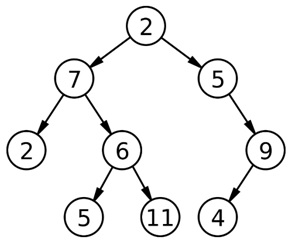# Find the number of leaf nodes in a Binary Tree | Data Structure

The article describes to find number of leaf nodes in a binary tree (C++ implementation).
Submitted by Radib Kar, on October 05, 2018

Algorithm:

One of the popular traversal techniques to solve this kind of problems is level order tree traversal (Read: Level Order Traversal on a Binary Tree) where we use the concept of BFS.

The basic idea to solve the problem is:

1. Do a level order traversal and check whether the processed node has its left and right child both NULL.
2. If the processed node has its left and right child both NULL, it’s a leaf node.
3. Increase leaf node count

Pseudocode:

```struct BT{              // tree node type
int data;           //value
struct BT *left;    //pointer to left child
struct BT *right;   //pointer to right child
};

int noofleafnodes(struct BT *root){ // root of the tree
// BT refers to node of tree (datatype for the node);
struct BT *temp;
struct Queue *q=Creat_queue();  //creating a queue
int count=0;
if(!root)   //root is null
return;
//**using level oder search**
EnQueue(q,root); // Enqueue the root in the queue

while(!emptyQueue(q)){
temp=DeQueue(q);  //Dequeue
// processing the node and checking whether both child nodes are NULL or not
if(!temp->left && !temp->right)
//increase no of leaves count if both child nodes are NULL (ensures it's a leaf  node)
count++;
else{
if(temp->left)
EnQueue(q,temp->left); // if left child exists EnQueue
if(temp->right)
EnQueue(q,temp->right); // if right child exists EnQueue
}
}
DeleteQueue(q);
return count; // return no of leaf nodes
}
```Image source: wikipedia

Example:

The leaf nodes in the above tree is 2, 5, 11, 4

C++ implementation:

```#include <bits/stdc++.h>
using namespace std;

class tree{    // tree node is defined
public:
int data;
tree *left;
tree *right;
};

int noofleafnodes( tree *root){
queue<tree*> q;  // using stl
tree* temp;
int count=0;
q.push(root);
while(!q.empty()){
temp=q.front();
q.pop();
//process node and check wheather leaf node or not
if(!temp->left && !temp->right){
count++;
cout<<temp->data<<" ";
}
if(temp->left)
q.push(temp->left); //EnQueue
if(temp->right)
q.push(temp->right); //EnQueue
}
cout<<endl;
return count;
}
tree* newnode(int data)  // creating new node
{
tree* node = (tree*)malloc(sizeof(tree));
node->data = data;
node->left = NULL;
node->right = NULL;

return(node);
}

int main()
{
//**same tree is builted as shown in example**
int c;
tree *root=newnode(2);
root->left= newnode(7);
root->right= newnode(5);
root->right->right=newnode(9);
root->right->right->left=newnode(4);
root->left->left=newnode(2);
root->left->right=newnode(6);
root->left->right->left=newnode(5);
root->left->right->right=newnode(11);

cout<<"printing the leaf nodes......"<<endl;
c=noofleafnodes(root);
cout<<"no of leaf nodes is : "<<c<<endl;

return 0;
}
```

Output

```printing the leaf nodes......
2 5 11 4
no of leaf nodes is : 4
```

Top MCQs

Languages: » C » C++ » C++ STL » Java » Data Structure » C#.Net » Android » Kotlin » SQL
Web Technologies: » PHP » Python » JavaScript » CSS » Ajax » Node.js » Web programming/HTML
Solved programs: » C » C++ » DS » Java » C#
Aptitude que. & ans.: » C » C++ » Java » DBMS
Interview que. & ans.: » C » Embedded C » Java » SEO » HR
CS Subjects: » CS Basics » O.S. » Networks » DBMS » Embedded Systems » Cloud Computing
» Machine learning » CS Organizations » Linux » DOS
More: » Articles » Puzzles » News/Updates